CATEGORIES:

# Bipartite graphs

A simple graph G is called bipartite if its vertex set V can be partitioned into two disjoint nonempty sets V1 and V2 such that every edge in the graph connects a vertex in V1 and a vertex in V2 (so that no edge in G connects either two vertices in V1 or two vertices in V2).

Example. C6 is bipartite since its vertex set can be partitioned into the two sets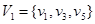and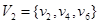, and every edge of C6 connects a vertex in V1 and a vertex in V2.

Example. K3 is not bipartite. To see this, note that if we divide the vertex set of K3 into two disjoint sets, one of the two sets must contain two vertices. If the graph were bipartite, these two vertices could not be connected by an edge, but in K3 each vertex is connected to every other vertex by an edge.

Example. Are the graphs G and H bipartite?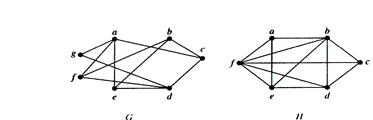Solution: Graph G is bipartite, since its vertex set is the union of two disjoint sets {a, b, d} and {c, e, f, g}, and each edge connects a vertex in one of these subsets to a vertex in the other subset. Note that for G to be bipartite it is not necessary that every vertex in {a, b, d} be adjacent to every vertex in {c, e, f, g}. For instance, b and g are not adjacent.

Graph H is not bipartite since its vertex set cannot be partitioned into two subsets so that edges do not connect two vertices from the same subset.

Example. The complete bipartite graph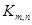is the graph that has its vertex set partitioned into two subsets of m and n vertices, respectively. There is an edge between two vertices iff one vertex is in the first subset and the other vertex is in the second subset.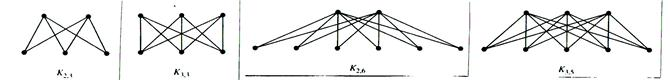Date: 2015-01-02; view: 1026

 <== previous page | next page ==> Some special simple graphs | Representing graphs
doclecture.net - lectures - 2014-2023 year. Copyright infringement or personal data (0.012 sec.)